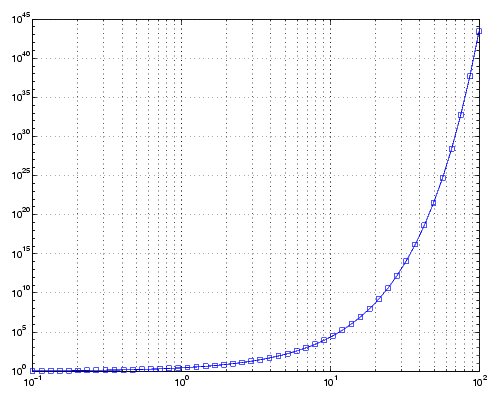MATLAB Function Referenceloglog

Log-log scale plot

Syntax

• ```loglog(Y)
loglog(X1,Y1,...)
loglog(X1,Y1,LineSpec,...)
loglog(...,'PropertyName',PropertyValue,...)
h = loglog(...)
hline = loglog('v6',...)
```

Description

```loglog(Y) ``` plots the columns of `Y` versus their index if `Y` contains real numbers. If `Y` contains complex numbers, `loglog(Y)` and `loglog(real(Y),imag(Y))` are equivalent. `loglog` ignores the imaginary component in all other uses of this function.

```loglog(X1,Y1,...) ``` plots all `Xn` versus `Yn` pairs. If only `Xn` or `Yn` is a matrix, `loglog` plots the vector argument versus the rows or columns of the matrix, depending on whether the vector's row or column dimension matches the matrix.

```loglog(X1,Y1,LineSpec,...) ``` plots all lines defined by the `Xn,Yn,LineSpec` triples, where `LineSpec` determines line type, marker symbol, and color of the plotted lines. You can mix `Xn,Yn,LineSpec` triples with `Xn,Yn` pairs, for example,

• ```loglog(X1,Y1,X2,Y2,`LineSpec`,X3,Y3)
```

```loglog(...,'PropertyName',PropertyValue,...) ``` sets property values for all lineseries graphics objects created by `loglog`. See the `line` reference page for more information.

```h = loglog(...) ``` returns a column vector of handles to lineseries graphics objects, one handle per line.

Backward Compatible Version

```hlines = loglog('v6',...) ``` returns the handles to line objects instead of lineseries objects.

Remarks

If you do not specify a color when plotting more than one line, `loglog` automatically cycles through the colors and line styles in the order specified by the current axes.

Examples

Create a simple `loglog` plot with square markers.

• ```x = logspace(-1,2);
loglog(x,exp(x),'-s')
grid on```

See Also

`LineSpec`, `plot`, `semilogx`, `semilogy`

Basic Plots and Graphs for related functionsLogical Operators: Short-circuit && || logm© 1994-2005 The MathWorks, Inc.